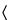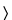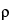699DENSITY OF SOLIDS

TERMS AND DEFINITIONS
Density refers to the average spatial distribution of mass in a material. The density of solids typically is expressed in g per cm3, in contrast to fluids, where the density is commonly expressed in g per mL at a stated reference temperature.
The density of a solid particle can assume different values depending on the method used to measure the volume of the particle. It is useful to distinguish among three different possibilities.
The true density of a substance is the average mass per unit volume, exclusive of all voids that are not a fundamental part of the molecular packing arrangement. It is a property of a particular material, and hence should be independent of the method of determination. The true density of a perfect crystal can be determined from the size and composition of the unit cell.
The pycnometric density, as measured by gas pycnometry, is a convenient density measurement for pharmaceutical powders. In a gas pycnometer, the volume occupied by a known mass of powder is determined by measuring the volume of gas displaced by the powder. The quotient of the mass and volume is the pycnometric density. The pycnometric density equals the true density unless the material contains impenetrable voids, or sealed pores, that are inaccessible to the gas used in the pycnometer.
The granular density includes contributions to particle volume from open pores smaller than some limiting size. The size limit depends on the method of measurement. A common measurement technique is mercury porosimetry, where the limiting pore size depends upon the maximum intrusion pressure. Because of the additional contribution from pore volume, the granular density will never be greater than the true density. A related concept is the aerodynamic density, which is the density of the particle with a volume defined by the aerodynamic envelope of the particle in a flowing stream. Both the closed and open pores contribute to this volume, but the open pores fill with the permeating fluid. The aerodynamic density, therefore, depends on the density of the test fluid if the particle is porous.
For brevity, the pycnometric density and the true density are both referred to as density. If needed, these quantities may be distinguished based on the method of measurement.
The density of a material depends on the molecular packing. For gases and liquids, the density will depend only on temperature and pressure. For solids, the density will also vary with the crystal structure and degree of crystallinity. If the solids are amorphous, the density may further depend upon the history of preparation and treatment. Therefore, unlike fluids, the densities of two chemically equivalent solids may be different, and this difference reflects a difference in solid-state structure. The density of constituent particles is an important physical characteristic of pharmaceutical powders.
Beyond these definitions of particle density, the bulk density of a powder includes the contribution of interparticulate void volume. Hence, the bulk density depends on both the density of powder particles and the packing of powder particles.

GAS PYCNOMETRY FOR THE MEASUREMENT OF DENSITY
Gas pycnometry is a convenient and suitable method for the measurement of the density of powder particles. A simple schematic of one type of gas pycnometer is shown in Figure 1.
Fig. 1. Schematic of Gas Pycnometer.
The sample, with mass w and volume Vs, is placed inside a sealed test cell with an empty cell volume of Vc. The system reference pressure, Pr, is determined at the manometer while the valve that connects the reference volume with the test cell is open. The valve is closed to separate the reference volume, Vr, from the test cell. The test cell is pressurized with the measurement gas to an initial pressure, Pi. Then the valve is opened to connect the reference volume, V r, with the test cell, and the pressure drops to the final pressure, Pf. If the measurement gas behaves ideally under the conditions of measurement, the sample volume, Vs, is given by the following expression:
The density,, is given by the equation:
Details of the instrumental design may differ, but all gas pycnometers rely on the measurement of pressure changes as a reference volume is added to, or deleted from, the test cell.
The measured density is a volume-weighted average of the densities of individual powder particles. The density will be in error if the test gas sorbs onto the powder or if volatile contaminants are evolved from the powder during the measurement. Sorption is prevented by an appropriate choice of test gas. Helium is the common choice. Volatile contaminants in the powder are removed by degassing the powder under a constant purge of helium prior to the measurement. Occasionally, powders may have to be degassed under vacuum. Two consecutive readings should yield sample volumes that are equal within 0.2% if volatile contaminants are not interfering with the measurements. Because volatiles may be evolved during the measurement, the weight of the sample should be taken after the pycnometric measurement of volume.
Method
Ensure that the reference volume and the calibration volume have been determined for the gas pycnometer by an appropriate calibration procedure. The test gas is helium, unless another gas is specified in the individual monograph. The temperature of the gas pycnometer should be between 15and 30and should not vary by more than 2during the course of the measurement. Load the test cell with the substance under examination that has been prepared according to the individual monograph. Where699Dis indicated, dry the substance under examination as directed for Loss on drying in the monograph unless other drying conditions are specified in the monograph Density of solids test. Where699Uis indicated, the substance under examination is used without drying. Use a quantity of powder recommended in the operating manual for the pycnometer. Seal the test cell in the pycnometer, and purge the pycnometer system with the test gas according to the procedure given in the manufacturer's operating instructions. If the sample must be degassed under vacuum, follow the recommendations in the individual monographs and the instructions in the operating manual for the pycnometer.
The measurement sequence above describes the procedure for the gas pycnometer shown in Figure 1. If the pycnometer differs in operation or in construction from the one shown in Figure 1, follow the operating procedure given in the manual for the pycnometer.
Repeat the measurement sequence for the same powder sample until consecutive measurements of the sample volume, Vs, agree to within 0.2%. Unload the test cell and measure the final powder weight, w. Calculate the pycnometric density,, of the sample according to Equation 2.

Auxiliary Information—